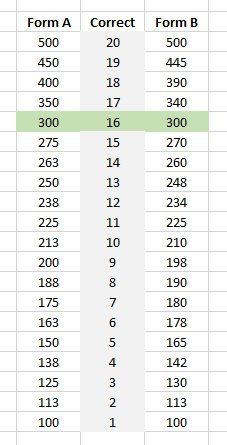# “300 out of 500” : What is a passing score?

All VMware certification exams have this listed in the blueprint:

The passing score for this exam is 300, using a scaled scoring method. The scale is from 100-500. Scaled scores are calculated using a mathematical formula that considers a variety of factors, including the number and type of exam questions included in a specific version of the exam. Because this combination may vary in different versions of the same examination, scaled scores provide a fair score for each individual based on the version of the exam taken.

But from what I’ve seen on social media most people seem to think this means they need to get either 60% (300/500) or 50% (300-100/500-100) of the questions correct. However, that would ignore most of what is in that paragraph. It also doesn’t address the wide variety of tests – how can there be exams with 23, 26,32, 85, or 135 questions and all have the same passing score?  They don’t! They have the same scaled passing score of 300.

For each exam a “cut score” or passing score is determined based on mathematical models from surveys, beta exams and other factors. Then a table is constructed where 0 points = 100, the passing score = 300 and all the points available = 500.

If an exam has 20 available points, and the passing score is determined to be 16, then getting no answers correct will earn a 100, getting 16 correct will be 300 and getting all 20 correct will result in a 500. Getting five correct should be around 150 but can fluctuate for the reasons stated and the fluctuations are per-form. For example:Note the “this combination may vary in different versions of the same examination” above. Depending on the questions presented on the exam, the table may change (the cut score is usually the same). So if two candidates take the exam at the same time but get different sets of questions and one scores a 175 the other a 180 did the second candidate get fewer correct answers? Only the psychometricians know.

tl/dr: The score you receive on the exam is mathematically sound and fair for your responses to the set of questions received. Comparison between candidates is not mathematically sound. Guessing how many correct answers are needed to pass is blind-man’s bluff.

The important thing to remember is to prepare well, have an excellent exam management strategy, and trust the math of the scoring.